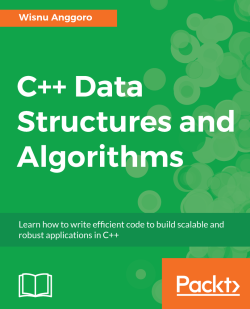### C++ Data Structures and Algorithms4.7 (7 reviews total)
By Wisnu Anggoro
• \$5 for 5 months Subscribe Access now
• £23.99 eBook
Advance your knowledge in tech with a Packt subscription

• Instant online access to over 7,500+ books and videos
• Constantly updated with 100+ new titles each month
• Breadth and depth in over 1,000+ technologies

#### About this book

C++ is a general-purpose programming language which has evolved over the years and is used to develop software for many different sectors. This book will be your companion as it takes you through implementing classic data structures and algorithms to help you get up and running as a confident C++ programmer.

We begin with an introduction to C++ data structures and algorithms while also covering essential language constructs. Next, we will see how to store data using linked lists, arrays, stacks, and queues. Then, we will learn how to implement different sorting algorithms, such as quick sort and heap sort. Along with these, we will dive into searching algorithms such as linear search, binary search and more. Our next mission will be to attain high performance by implementing algorithms to string datatypes and implementing hash structures in algorithm design. We'll also analyze Brute Force algorithms, Greedy algorithms, and more.

By the end of the book, you'll know how to build components that are easy to understand, debug, and use in different applications.

Publication date:
April 2018
Publisher
Packt
Pages
322
ISBN
9781788835213

## Chapter 1. Learning Data Structures and Algorithms in C++

In this first chapter, we are going to build the solid foundations so we can go through the following chapters easily. The topics we are going to discuss in this chapter are:

• Creating, building, and running a simple C++ program
• Constructing an abstract data type to make a user-defined data type
• Leveraging the code with C++ templates and the Standard Template Library (STL)
• Analyzing the complexity of algorithms to measure the performance of the code

## Technical requirements

To follow along with this chapter including the source code, we require the following:

## Introduction to basic C++

Before we go through the data structures and algorithms in C++, we need to have a strong, fundamental understanding of the language itself. In this section, we are going to build a simple program, build it, and then run it. We are also going to discuss the fundamental and advanced data types, and before we move on to algorithm analysis, we are going to discuss control flow in this section.

### Creating your first code in C++

In C++, the code is executed from the `main()` function first. The function itself is a collection of statements to perform a specific task. As a result of this, a program in C++ has to contain at least one function named `main()`. The following code is the simplest program in C++ that will be successfully compiled and run:

```    int main()
{
return 0;
}```

Suppose the preceding code is saved as a `simple.cpp` file. We can compile the code using the g++ compiler by running the following compiling command on the console from the active directory where the `simple.cpp` file is placed:

`g++ simple.cpp`

If no error message appears, the output file will be generated automatically. If we run the preceding compiling command on a Windows console, we will get a file named `a.exe`. However, if we run the command on Bash shells, such as Linux or macOS, we will get a file named `a.out`.

We can specify the output file name using the `-o` option followed by the desired filename. For instance, the following compiling command will produce the output file named `simple.out`:

`g++ simple.cpp -o simple.out`

Indeed, when we run the output file (by typing `a` and then pressing Enter on a Windows console or by typing `./a.out` and then pressing Enter on Bash shell), we won't see anything on the console window. This is because we don't print anything to the console yet. To make our `simple.cpp` file meaningful, let's refactor the code so that it can receive the input data from the user and then print the data back to the user. The refactored code should look as follows:

```    // in_out.cpp
#include <iostream>

int main ()
{
int i;
std::cout << "Please enter an integer value: ";
std::cin >> i;
std::cout << "The value you entered is " << i;
std::cout << "\n";
return 0;
}```

As we can see in the preceding code, we appended several lines of code so that the program can print to the console and the user can give an input to the program. Initially, the program displays text that asks the user to input an integer number. After the user types the desired number and presses Enter, the program will print that number. We also defined a new variable named `i` of the `int` data type. This variable is used to store data in an integer data format (we will talk about variables and data types in the upcoming section).

Suppose we save the preceding code as `in_out.cpp`; we can compile it using the following command:

`g++ in_out.cpp`

If we then run the program, we will get the following output on the console (I chose the number `3` in this example):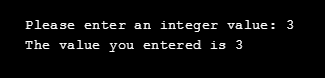Now, we know that to print text to the console, we use the `std::cout` command, and to give some inputs to the program, we use the `std::cin` command. In the `in_out.cpp` file, we also see `#include <iostream>` at the beginning of the file. It's used to tell the compiler where to find the implementation of the `std::cout` and `std::cin` commands since their implementation is stated in the `iostream` header file.

And at the very beginning of the file, we can see that the line begins with double slashes (`//`). This means that the line won't be considered as code, so the compiler will ignore it. It's used to comment or mark an action in the code so that other programmers can understand our code.

### Enhancing code development experience with IDE

So far, we have been able to create a C++ code, compile the code, and run the code. However, it will be boring if we have to compile the code using the Command Prompt and then execute the code afterwards. To ease our development process, we will use an integrated development environment (IDE) so that we can compile and run the code with just a click. You can use any C++ IDE available on the market, either paid or free. However, I personally chose Code::Blocks IDE since it's free, open source, and cross-platform so it can run on Windows, Linux, and macOS machines. You can find the information about this IDE, such as how to download, install, and use it on its official website at http://www.codeblocks.org/.

Actually, we can automate the compiling process using a toolchain such as Make or CMake. However, this needs further explanation, and since this book is intended to discuss data structures and algorithms, the toolchain explanation will increase the total pages of the book, and so we will not discuss this here. In this case, the use of IDE is the best solution to automate the compiling process since it actually accesses the toolchain as well.

After installing Code::Blocks IDE, we can create a new project by clicking on the `File` menu, then clicking `New`, and then selecting `Project`. A new window will appear and we can select the desired project type. For most examples in this book, we will use the `Console Application` as the project type. Press the `Go` button to continue.

On the upcoming window, we can specify the language, which is C++, and then define the project name and destination location (I named the project `FirstApp`). After finishing the wizard, we will have a new project with a `main.cpp` file containing the following code:

```    #include <iostream>

using namespace std;

int main()
{
cout << "Hello world!" << endl;
return 0;
}```

Now, we can compile and run the preceding code by just clicking the `Build and run` option under the `Build` menu. The following console window will appear: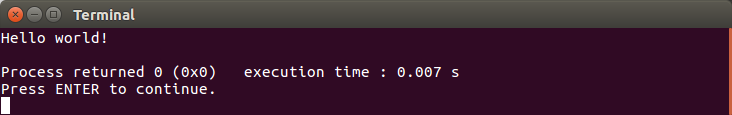In the preceding screenshot, we see the console using `namespace std` in the line after the `#include <iostream>` line. The use of this line of code is to tell the compiler that the code uses a `namespace` named `std`. As a result, we don't need to specify the `std::` in every invocation of the `cin` and `cout` commands. The code should be simpler than before.

### Defining the variables using fundamental data types

In the previous sample codes, we dealt with the variable (a placeholder is used to store a data element) so that we can manipulate the data in the variable for various operations. In C++, we have to define a variable to be of a specific data type so it can only store the specific type of variable that was defined previously. Here is a list of the fundamental data types in C++. We used some of these data types in the previous example:

• Boolean data type (`bool`), which is used to store two pieces of conditional data only—`true` or `false`
• Character data type (`char``wchar_t``char16_t`, and `char32_t`), which is used to store a single ASCII character
• Floating point data type (`float``double`, and` long double`), which is used to store a number with a decimal
• Integer data type (`short``int``long`, and `long long`), which is used to store a whole number
• No data (`void`), which is basically a keyword to use as a placeholder where you would normally put a data type to represent no data

There are two ways to create a variable—by defining it or by initializing it. Defining a variable will create a variable without deciding upon its initial value. The initializing variable will create a variable and store an initial value in it. Here is the code snippet for how we can define variables:

```    int iVar;
char32_t cVar;
long long llVar;
bool boVar;```

And here is the sample code snippet of how initializing variables works:

```    int iVar = 100;
char32_t cVar = 'a';
long long llVar = 9223372036854775805;
bool boVar = true;```

The preceding code snippet is the way we initialize the variables using the copy initialization technique. In this technique, we assign a value to the variable using an equals sign symbol (`=`). Another technique we can use to initialize a variable is the direct initialization technique. In this technique, we use parenthesis to assign a value to the variable. The following code snippet uses this technique to initialize the variables:

```    int iVar(100);
char32_t cVar('a');
long long llVar(9223372036854775805);
bool boVar(true);```

Besides copy initialization and direct initialization techniques, we can use uniform initialization by utilizing curly braces. The following code snippet uses the so-called brace-initialization technique to initialize the variables:

```    int iVar{100};
char32_t cVar{'a'};
long long llVar{9223372036854775805};
bool boVar{true};```

We cannot define a variable with a `void` data type such as void `vVar` because when we define a variable, we have to decide what data type we are choosing so that we can store the data in the variable. If we define a variable as `void`, it means that we don't plan to store anything in the variable.

### Controlling the flow of the code

As we discussed earlier, the C++ program is run from the `main()` function by executing each statement one by one from the beginning to the end. However, we can change this path using flow control statements. There are several flow control statements in C++, but we are only going to discuss some of them, since these are the ones that are going to be used often in algorithm design.

#### Conditional statement

One of the things that can make the flow of a program change is a conditional statement. By using this statement, only the line in the `true` condition will be run. We can use the `if` and `else` keywords to apply this statement.

Let's modify our previous `in_out.cpp` code so that it uses the conditional statement. The program will only decide whether the input number is greater than `100` or not. The code should be as follows:

```    // If.cbp
#include <iostream>

using namespace std;

int main ()
{
int i;
cout << "Please enter an integer value: ";
cin >> i;
cout << "The value you entered is ";

if(i > 100)
cout << "greater than 100.";
else
cout << "equals or less than 100.";

cout << endl;
return 0;
}```

As we can see, we have a pair of the `if` and `else` keywords that will decide whether the input number is greater than `100` or not. By examining the preceding code, only one statement will be executed inside the conditional statement, either the statement under the `if` keyword or the statement under the `else` keyword.

If we build and run the preceding code, we will see the following console window: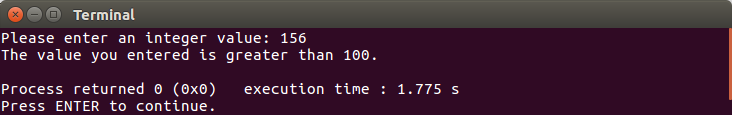From the preceding console window, we can see that the line `std::cout << "equals or less than 100.";` is not executed at all since we have input a number that is greater than `100`.

Also, in the `if...else` condition, we can have more than two conditional statements. We can refactor the preceding code so that it has more than two conditional statements, as follows:

```    // If_ElseIf.cbp
#include <iostream>

using namespace std;

int main ()
{
int i;
cout << "Please enter an integer value: ";
cin >> i;
cout << "The value you entered is ";

if(i > 100)
cout << "greater than 100.";
else if(i < 100)
cout << "less than 100.";
else
cout << "equals to 100";

cout << endl;
return 0;
}```

Another conditional statement keyword is `switch`. Before we discuss this keyword, let's create a simple calculator program that can add two numbers. It should also be capable of performing the subtract, multiply, and divide operations. We will use the `if...else` keyword first. The code should be as follows:

```    // If_ElseIf_2.cbp
#include <iostream>

using namespace std;

int main ()
{
int i, a, b;

cout << "Operation Mode: " << endl;
cout << "1. Addition" << endl;
cout << "2. Subtraction" << endl;
cout << "3. Multiplication" << endl;
cout << "4. Division" << endl;
cout << "Please enter the operation mode: ";
cin >> i;

cout << "Please enter the first number: ";
cin >> a;
cout << "Please enter the second number: ";
cin >> b;

cout << "The result of ";

if(i == 1)
cout << a << " + " << b << " is " << a + b;
else if(i == 2)
cout << a << " - " << b << " is " << a - b;
else if(i == 3)
cout << a << " * " << b << " is " << a * b;
else if(i == 4)
cout << a << " / " << b << " is " << a / b;

cout << endl;
return 0;
}```

As we can see in the preceding code, we have four options that we have to choose from. We use the `if...else` conditional statement for this purpose. The output of the preceding code should be as follows: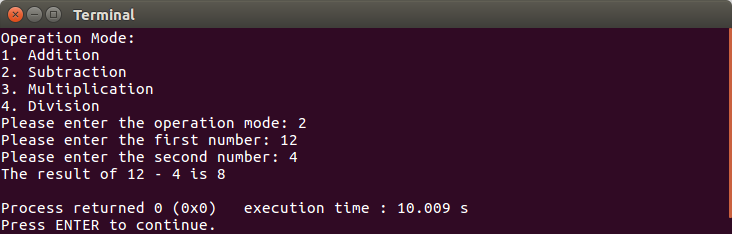However, we can use the `switch` keyword as well. The code should be as follows after being refactored:

```    // Switch.cbp
#include <iostream>

using namespace std;

int main ()
{
int i, a, b;

cout << "Operation Mode: " << endl;
cout << "1. Addition" << endl;
cout << "2. Subtraction" << endl;
cout << "3. Multiplication" << endl;
cout << "4. Division" << endl;
cout << "Please enter the operation mode: ";
cin >> i;

cout << "Please enter the first number: ";
cin >> a;
cout << "Please enter the second number: ";
cin >> b;

cout << "The result of ";

switch(i)
{
case 1:
cout << a << " + " << b << " is " << a + b;
break;
case 2:
cout << a << " - " << b << " is " << a - b;
break;
case 3:
cout << a << " * " << b << " is " << a * b;
break;
case 4:
cout << a << " / " << b << " is " << a / b;
break;
}

cout << endl;
return 0;
}```

And if we run the preceding code, we will get the same output compared with the `If_ElseIf_2.cbp` code.

#### Loop statement

There are several loop statements in C++, and they are `for``while`, and `do...while`. The `for` loop is usually used when we know how many iterations we want, whereas `while` and `do...while` will iterate until the desired condition is met.

Suppose we are going to generate ten random numbers between `0` to `100`; the `for` loop is the best solution for it since we know how many numbers we need to generate. For this purpose, we can create the following code:

```// For.cbp
#include <iostream>
#include <cstdlib>
#include <ctime>

using namespace std;

int GenerateRandomNumber(int min, int max)
{
// static used for efficiency,
// so we only calculate this value once
static const double fraction =
1.0 / (static_cast<double>(RAND_MAX) + 1.0);

// evenly distribute the random number
// across our range
return min + static_cast<int>(
(max - min + 1) * (rand() * fraction));
}

int main()
{
// set initial seed value to system clock
srand(static_cast<unsigned int>(time(0)));

// loop ten times
for (int i=0; i < 10; ++i)
{

cout << GenerateRandomNumber(0, 100) << " ";
}
cout << "\n";

return 0;
}```

From the preceding code, we create another function besides `main()`, that is, `GenerateRandomNumber()`. The code will invoke the function ten times using the `for` loop, as we can see in the preceding code. The output we will get should be as follows: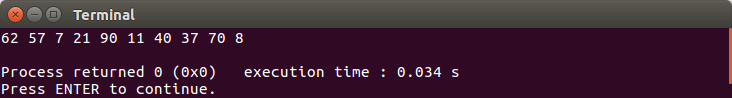Back to the others loop statements which we discussed earlier, which are `while` and `do...while` loop. They are quite similar based on their behavior. The difference is when we use the `while` loop, there is a chance the statement inside the loop scope is not run, whereas the statement in the loop scope must be run at least once in the `do...while` loop.

Now, let's create a simple game using those loop statements. The computer will generate a number between `1` to `100`, and then the user has to guess what number has been generated by the computer. The program will give a hint to the user just after she or he inputs the guessed number. It will tell the user whether the number is greater than the computer's number or lower than it. If the guessed number matches with the computer's number, the game is over. The code should be as follows:

```// While.cbp
#include <iostream>
#include <cstdlib>
#include <ctime>

using namespace std;

int GenerateRandomNumber(int min, int max)
{
// static used for efficiency,
// so we only calculate this value once
static const double fraction =
1.0 / (static_cast<double>(RAND_MAX) + 1.0);

// evenly distribute the random number
// across our range
return min + static_cast<int>(
(max - min + 1) * (rand() * fraction));
}

int main()
{
// set initial seed value to system clock
srand(static_cast<unsigned int>(time(0)));

// Computer generate random number
// between 1 to 100
int computerNumber = GenerateRandomNumber(1, 100);

// User inputs a guessed number
int userNumber;
cout << "Please enter a number between 1 to 100: ";
cin >> userNumber;

// Run the WHILE loop
while(userNumber != computerNumber)
{
cout << userNumber << " is ";
if(userNumber > computerNumber)
cout << "greater";
else
cout << "lower";
cout << " than computer's number" << endl;
cout << "Choose another number: ";
cin >> userNumber;
}

cout << "Yeeaayy.. You've got the number." << endl;
return 0;
}```

From the preceding code, we can see that we have two variables, `computerNumber` and `userNumber`, handling the number that will be compared. There will be a probability that `computerNumber` is equal to `userNumber`. If this happens, the statement inside the `while` loop scope won't be executed at all. The flow of the preceding program can be seen in the following output console screenshot: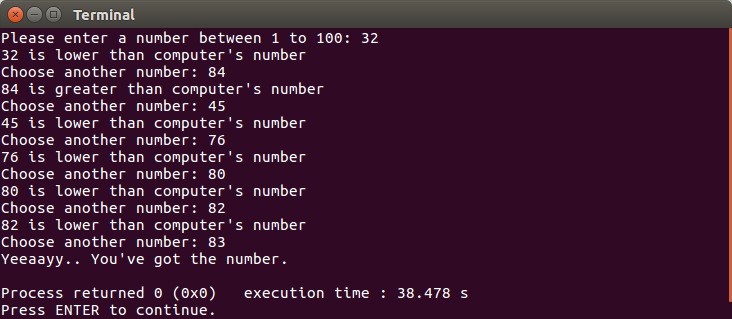We have successfully implemented the `while` loop in the preceding code. Although the `while` loop is similar to the `do...while` loop, as we discussed earlier, we cannot refactor the preceding code by just replacing the `while` loop with the `do...while` loop. However, we can create another game as our example in implementing the `do...while` loop. However, now the user has to choose a number and then the program will guess it. The code should be as follows:

```// Do-While.cbp
#include <iostream>
#include <cstdlib>
#include <ctime>

using namespace std;

int GenerateRandomNumber(int min, int max)
{
// static used for efficiency,
// so we only calculate this value once
static const double fraction =
1.0 / (static_cast<double>(RAND_MAX) + 1.0);

// evenly distribute the random number
// across our range
return min + static_cast<int>(
(max - min + 1) * (rand() * fraction));
}

int main()
{
// set initial seed value to system clock
srand(static_cast<unsigned int>(time(0)));

char userChar;

int iMin = 1;
int iMax = 100;
int iGuess;

// Menu display
cout << "Choose a number between 1 to 100, ";
cout << "and I'll guess your number." << endl;
cout << "Press L and ENTER if my guessed number is lower than
yours";
cout << endl;
cout << "Press G and ENTER if my guessed number is greater than
yours";
cout << endl;
cout << "Press Y and ENTER if I've successfully guessed your
number!";
cout << endl << endl;

// Run the DO-WHILE loop
do
{
iGuess = GenerateRandomNumber(iMin, iMax);
cout << "I guess your number is " << iGuess << endl;
cout << "What do you think? ";
cin >> userChar;
if(userChar == 'L' || userChar == 'l')
iMin = iGuess + 1;
else if(userChar == 'G' || userChar == 'g')
iMax = iGuess - 1;

}
while(userChar != 'Y' && userChar != 'y');

cout << "Yeeaayy.. I've got your number." << endl;
return 0;
}```

As we can see in the preceding code, the program has to guess the user's number at least once, and if it's lucky, the guessed number matches with the user's number, so that we use the `do...while` loop here. When we build and run the code, we will have an output similar to the following screenshot: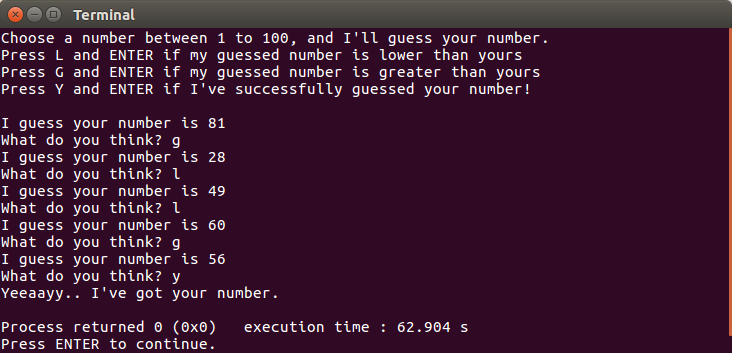In the preceding screenshot, I chose number `56`. The program then guessed `81`. Since the number is greater than my number, the program guessed another number, which is `28`. It then guessed another number based on the hint from me until it found the correct number. The program will leave the `do...while` loop if the user presses `y`, as we can see in the preceding code.

### Leveraging the variable capability using advanced data types

We have discussed the fundamental data type in the previous section. This data type is used in defining or initializing a variable to ensure that the variable can store the selected data type. However, there are other data types that can be used to define a variable. They are enum (enumeration) and struct.

Enumeration is a data type that has several possible values and they are defined as the constant which is called enumerators. It is used to create a collection of constants. Suppose we want to develop a card game using C++. As we know, a deck of playing cards contains 52 cards, which consists of four suits (Clubs, Diamonds, Hearts, and Spades) with 13 elements in each suit. We can notate the card deck as follows:

```enum CardSuits
{
Club,
Diamond,
Heart,
Spade
};

enum CardElements
{
Ace,
Two,
Three,
Four,
Five,
Six,
Seven,
Eight,
Nine,
Ten,
Jack,
Queen,
King
};```

If we want to apply the preceding `enum` data types (`CardSuits` and `CardElements`), we can use the following variable initialization:

```CardSuits suit = Club;
CardElements element = Ace;```

Actually, enums always contain integer constants. The string we put in the `enum` element is the constant name only. The first element holds a value of `0`, except we define another value explicitly. The next elements are in an incremental number from the first element. So, for our preceding `CardSuits` enum, `Club` is equal to `0`, and the `Diamond`, `Heart`, and `Spade` are `1`, `2`, and `3`, respectively.

Now, let's create a program that will generate a random card. We can borrow the `GenerateRandomNumber()` function from our previous code. The following is the complete code for this purpose:

```// Enum.cbp
#include <iostream>
#include <cstdlib>
#include <ctime>

using namespace std;

enum CardSuits
{
Club,
Diamond,
Heart,
Spade
};

enum CardElements
{
Ace,
Two,
Three,
Four,
Five,
Six,
Seven,
Eight,
Nine,
Ten,
Jack,
Queen,
King
};

string GetSuitString(CardSuits suit)
{
string s;

switch(suit)
{
case Club:
s = "Club";
break;
case Diamond:
s = "Diamond";
break;
case Heart:
s = "Heart";
break;
case Spade:
s = "Spade";
break;
}

return s;
}

string GetElementString(CardElements element)
{
string e;

switch(element)
{
case Ace:
e = "Ace";
break;
case Two:
e = "Two";
break;
case Three:
e = "Three";
break;
case Four:
e = "Four";
break;
case Five:
e = "Five";
break;
case Six:
e = "Six";
break;
case Seven:
e = "Seven";
break;
case Eight:
e = "Eight";
break;
case Nine:
e = "Nine";
break;
case Ten:
e = "Ten";
break;
case Jack:
e = "Jack";
break;
case Queen:
e = "Queen";
break;
case King:
e = "King";
break;
}

return e;
}

int GenerateRandomNumber(int min, int max)
{
// static used for efficiency,
// so we only calculate this value once
static const double fraction =
1.0 / (static_cast<double>(RAND_MAX) + 1.0);

// evenly distribute the random number
// across our range
return min + static_cast<int>(
(max - min + 1) * (rand() * fraction));
}

int main()
{
// set initial seed value to system clock
srand(static_cast<unsigned int>(time(0)));

// generate random suit and element card
int iSuit = GenerateRandomNumber(0, 3);
int iElement = GenerateRandomNumber(0, 12);

CardSuits suit = static_cast<CardSuits>(
iSuit);
CardElements element = static_cast<CardElements>(
iElement);

cout << "Your card is ";
cout << GetElementString(element);
cout << " of " << GetSuitString(suit) << endl;

return 0;
}```

From the preceding code, we can see that we can access the `enum` data by using an integer value. However, we have to cast the `int` value so that it can fit the `enum` data by using `static_cast<>`, which is shown as follows:

```int iSuit = GenerateRandomNumber(0, 3);
int iElement = GenerateRandomNumber(0, 12);

CardSuits suit = static_cast<CardSuits>(iSuit);
CardElements element = static_cast<CardElements>(iElement);```

If we build and run the code, we will get the following console output: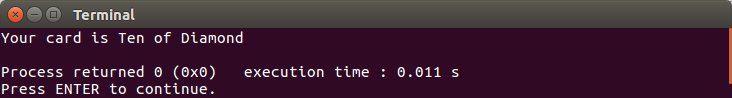Another advanced data type we have in C++ is structs. It is an aggregate data type which groups multiple individual variables together. From the preceding code, we have the `suit` and `element` variables that can be grouped as follows:

```struct Cards
{
CardSuits suit;
CardElements element;
};```

If we add the preceding `struct` to our preceding `Enum.cbp` code, we just need to refactor the `main()` function as follows:

```int main()
{
// set initial seed value to system clock
srand(static_cast<unsigned int>(time(0)));

Cards card;
card.suit = static_cast<CardSuits>(
GenerateRandomNumber(0, 3));
card.element = static_cast<CardElements>(
GenerateRandomNumber(0, 12));

cout << "Your card is ";
cout << GetElementString(card.element);
cout << " of " << GetSuitString(card.suit) << endl;

return 0;
}```

If we run the preceding code (you can find the code as `Struct.cbp` in the repository), we will get the same output as `Enum.cbp`.

## Developing abstract data types

An abstract data type (ADT) is a type that consists of a collection of data and associated operations for manipulating the data. The ADT will only mention the list of operations that can be performed but not the implementation. The implementation itself is hidden, which is why it's called abstract.

Imagine we have a DVD player we usually use in our pleasure time. The player has a remote control, too. The remote control has various menu buttons such as ejecting the disc, playing or stopping the video, increasing or decreasing volume, and so forth. Similar to the ADT, we don't have any idea how the player increases the volume when we press the increasing button (similar to the operation in ADT). We just call the increasing operation and then the player does it for us; we do not need to know the implementation of that operation.

Regarding the process flow, we need to take into account the ADT's implement abstraction, information hiding, and encapsulation techniques. The explanation of these three techniques is as follows:

• Abstraction is hiding the implementation details of the operations that are available in the ADT
• Information hiding is hiding the data which is being affected by that implementation
• Encapsulation is grouping all similar data and functions into a group

### Applying C++ classes to build user-defined ADTs

Classes are containers for variables and the operations (methods) that will affect the variables. As we discussed earlier, as ADTs implement encapsulation techniques for grouping all similar data and functions into a group, the classes can also be applied to group them. A class has three access control sections for wrapping the data and methods, and they are:

• Public: Data and methods can be accessed by any user of the class
• Protected: Data and methods can only be accessed by class methods, derived classes, and friends
• Private: Data and methods can only be accessed by class methods and friends

Let's go back to the definition of abstraction and information hiding in the previous section. We can implement abstraction by using `protected` or `private` keywords to hide the methods from outside the class and implement the information hiding by using a `protected` or `private` keyword to hide the data from outside the class.

Now, let's build a simple class named `Animal`, as shown in the following code snippet:

```class Animal
{
private:
string m_name;

public:
void GiveName(string name)
{
m_name = name;
}

string GetName()
{
return m_name;
}
};```

As we can see in the preceding code snippet, we cannot access the `m_name` variable directly since we assigned it as `private`. However, we have two `public` methods to access the variable from the inside class. The `GiveName()` methods will modify the `m_name` value, and the `GetName()` methods will return the `m_name` value. The following is the code to consume the `Animal` class:

```// Simple_Class.cbp
#include <iostream>

using namespace std;

class Animal
{
private:
string m_name;

public:
void GiveName(string name)
{
m_name = name;
}

string GetName()
{
return m_name;
}
};

int main()
{
Animal dog = Animal();
dog.GiveName("dog");

cout << "Hi, I'm a " << dog.GetName() << endl;

return 0;
}```

In the preceding code, we created a variable named `dog` of the type `Animal`. Since then, the `dog` has the ability that `Animal` has, such as invoking the `GiveName()` and `GetName()` methods. The following is the window we should see if we build and run the code: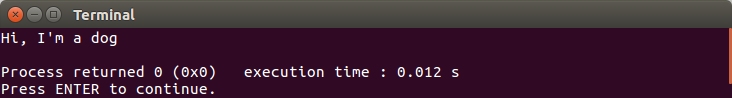Now, we can say that `Animal` ADT has two functions, and they are `GiveName(string name)` and `GetName()`.

After discussing simple class, you might see that there's a similarity between structs and classes. They both actually have similar behaviors. The differences are, however, that structs have the default `public` members, while classes have the default `private` members. I personally recommend using structs as data structures only (they don't have any methods in them) and using classes to build the ADTs.

As we can see in the preceding code, we assign the variable to the instance of the `Animal` class by using its constructor, which is shown as follows:

`Animal dog = Animal();`

However, we can initialize a class data member by using a class constructor. The constructor name is the same as the class name. Let's refactor our preceding `Animal` class so it has a constructor. The refactored code should be as follows:

```// Constructor.cbp
#include <iostream>

using namespace std;

class Animal
{
private:
string m_name;

public:
Animal(string name) : m_name(name)
{

}

string GetName()
{
return m_name;
}
};

int main()
{
Animal dog = Animal("dog");

cout << "Hi, I'm a " << dog.GetName() << endl;

return 0;
}```

As we can see in the preceding code, when we define the `dog` variable, we also initialize the `m_name` private variable of the class. We don't need the `GiveName()` method anymore to assign the `private` variable. Indeed, we will get the same output again if we build and run the preceding code.

In the preceding code, we assign `dog` as the `Animal` data type. However, we can also derive a new class based on the base class. By deriving from the base class, the derived class will also have the behavior that the base class has. Let's refactor the `Animal` class again. We will add a virtual method named `MakeSound()`. The virtual method is a method that has no implementation yet, and only has the definition (also known as the interface). The derived class has to add the implementation to the virtual method using the `override` keyword, or else the compiler will complain. After we have a new `Animal` class, we will make a class named `Dog` that derives from the `Animal` class. The code should be as follows:

```// Derived_Class.cbp
#include <iostream>

using namespace std;

class Animal
{
private:
string m_name;

public:
Animal(string name) : m_name(name)
{

}

// The interface that has to be implemented
// in derived class
virtual string MakeSound() = 0;

string GetName()
{
return m_name;
}
};

class Dog : public Animal
{
public:
// Forward the constructor arguments
Dog(string name) : Animal(name) {}

// here we implement the interface
string MakeSound() override
{
return "woof-woof!";
}
};

int main()
{
Dog dog = Dog("Bulldog");

cout << dog.GetName() << " is barking: ";
cout << dog.MakeSound() << endl;

return 0;
}```

Now, we have two classes, the `Animal` class (as the base class) and the `Dog` class (as the derived class). As shown in the preceding code, the `Dog` class has to implement the `MakeSound()` method since it has been defined as a virtual method in the `Animal` class. The instance of the `Dog` class can also invoke the `GetName()` method, even though it's not implemented inside the `Dog` class since the derived class derives all base class behavior. If we run the preceding code, we will see the following console window: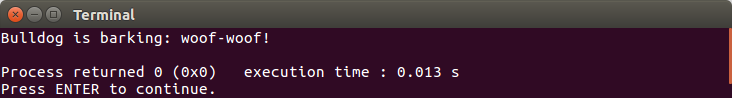Again, we can say that the `Dog` ADT has two functions, and they are the `GetName()` and `MakeSound()` functions.

Another necessary requirement of ADT is that it has to be able to control all copy operations to avoid dynamic memory aliasing problems caused by shallow copying (some members of the copy may reference the same objects as the original). For this purpose, we can use assignment operator overloading. As the sample, we will refactor the `Dog` class so it now has the `copy assignment` operator. The code should be as follows:

```// Assignment_Operator_Overload.cbp
#include <iostream>

using namespace std;

class Animal
{
protected:
string m_name;

public:
Animal(string name) : m_name(name)
{

}

// The interface that has to be implemented
// in derived class
virtual string MakeSound() = 0;

string GetName()
{
return m_name;
}
};

class Dog : public Animal
{
public:
// Forward the constructor arguments
Dog(string name) : Animal(name) {}

// Copy assignment operator overloading
void operator = (const Dog &D)
{
m_name = D.m_name;
}

// here we implement the interface
string MakeSound() override
{
return "woof-woof!";
}
};

int main()
{
Dog dog = Dog("Bulldog");
cout << dog.GetName() << " is barking: ";
cout << dog.MakeSound() << endl;

Dog dog2 = dog;
cout << dog2.GetName() << " is barking: ";
cout << dog2.MakeSound() << endl;

return 0;
}```

We have added a `copy assignment` operator which is overloading in the `Dog` class. However, since we tried to access the `m_name` variable in the base class from the derived class, we need to make `m_name``protected` instead of `private`. In the `main()` method, when we copy `dog` to `dog2` in `Dog dog2 = dog;`, we can ensure that it's not a shallow copy.

### Playing with templates

Now, let's play with the templates. The templates are the features that allow the functions and classes to operate with generic types. It makes a function or class work on many different data types without having to be rewritten for each one. Using the template, we can build various data types, which we will discuss later in this book.

#### Function templates

Suppose we have another class that is derived from the `Animal` class, for instance, `Cat`. We are going to make a function that will invoke the `GetName()` and `MakeSound()` methods for both the `Dog` and `Cat` instances. Without creating two separated functions, we can use the template, which is shown as follows:

```// Function_Templates.cbp
#include <iostream>

using namespace std;

class Animal
{
protected:
string m_name;

public:
Animal(string name) : m_name(name)
{

}

// The interface that has to be implemented
// in derived class
virtual string MakeSound() = 0;

string GetName()
{
return m_name;
}
};

class Dog : public Animal
{
public:
// Forward the constructor arguments
Dog(string name) : Animal(name) {}

// Copy assignment operator overloading
void operator = (const Dog &D)
{
m_name = D.m_name;
}

// here we implement the interface
string MakeSound() override
{
return "woof-woof!";
}
};

class Cat : public Animal
{
public:
// Forward the constructor arguments
Cat(string name) : Animal(name) {}

// Copy assignment operator overloading
void operator = (const Cat &D)
{
m_name = D.m_name;
}

// here we implement the interface
string MakeSound() override
{
return "meow-meow!";
}
};

template<typename T>
void GetNameAndMakeSound(T& theAnimal)
{
cout << theAnimal.GetName() << " goes ";
cout << theAnimal.MakeSound() << endl;
}

int main()
{
Dog dog = Dog("Bulldog");
GetNameAndMakeSound(dog);

Cat cat = Cat("Persian Cat");
GetNameAndMakeSound(cat);

return 0;
}```

From the preceding code, we can see that we can pass both the `Dog` and `Cat` data types to the `GetNameAndMakeSound()` function since we have defined the `<typename T>` template before the function definition. The `typename` is a keyword in C++, which is used to write a template. The keyword is used for specifying that a symbol in a template definition or declaration is a type (in the preceding example, the symbol is `T`). As a result, the function becomes generic and can accept various data types, and if we build and run the preceding code, we will be shown the following console window: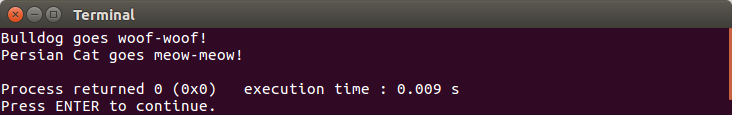Please ensure that the data type we pass to the generic function has the ability to do all the operation invoking from the generic function. However, the compiler will compile if the data type we pass does not have the expected operation. In the preceding function template example, we need to pass a data type that is an instance of the `Animal` class, so we can pass either instance of the `Animal` class or an instance of a derived class of the `Animal` class.

#### Class templates

Similar to the function template, the class template is used to build a generic class that can accept various data types. Let's refactor the preceding `Function_Template.cbp` code by adding a new class template. The code should be as follows:

```// Class_Templates.cbp
#include <iostream>

using namespace std;

class Animal
{
protected:
string m_name;

public:
Animal(string name) : m_name(name)
{

}

// The interface that has to be implemented
// in derived class
virtual string MakeSound() = 0;

string GetName()
{
return m_name;
}
};

class Dog : public Animal
{
public:
// Forward the constructor arguments
Dog(string name) : Animal(name) {}

// Copy assignment operator overloading
void operator = (const Dog &D)
{
m_name = D.m_name;
}

// here we implement the interface
string MakeSound() override
{
return "woof-woof!";
}
};

class Cat : public Animal
{
public:
// Forward the constructor arguments
Cat(string name) : Animal(name) {}

// Copy assignment operator overloading
void operator = (const Cat &D)
{
m_name = D.m_name;
}

// here we implement the interface
string MakeSound() override
{
return "meow-meow!";
}

};

template<typename T>
void GetNameAndMakeSound(T& theAnimal)
{
cout << theAnimal.GetName() << " goes ";
cout << theAnimal.MakeSound() << endl;
}

template <typename T>
class AnimalTemplate
{
private:
T m_animal;

public:
AnimalTemplate(T animal) : m_animal(animal) {}

void GetNameAndMakeSound()
{
cout << m_animal.GetName() << " goes ";
cout << m_animal.MakeSound() << endl;
}
};

int main()
{
Dog dog = Dog("Bulldog");
AnimalTemplate<Dog> dogTemplate(dog);
dogTemplate.GetNameAndMakeSound();

Cat cat = Cat("Persian Cat");
AnimalTemplate<Cat> catTemplate(cat);
catTemplate.GetNameAndMakeSound();

return 0;
}```

As we can see in the preceding code, we have a new class named `AnimalTemplate`. It's a template class and it can be used by any data type. However, we have to define the data type in the angle bracket, as we can see in the preceding code, when we use the instances `dogTemplate` and `catTemplate`. If we build and run the code, we will get the same output as we did for the `Function_Template.cbp` code.

#### Standard Template Library

C++ programming has another powerful feature regarding the use of template classes, which is the Standard Template Library. It's a set of class templates to provide all functions that are commonly used in manipulating various data structures. There are four components that build the STL, and they are algorithms, containers, iterators, and functions. Now, let's look at these components further.

Algorithms are used on ranges of elements, such as sorting and searching. The sorting algorithm is used to arrange the elements, both in ascending and descending order. The searching algorithm is used to look for a specific value from the ranges of elements.

Containers are used to store objects and data. The common container that is widely used is vector. The vector is similar to an array, except it has the ability to resize itself automatically when an element is inserted or deleted.

Iterators are used to work upon a sequence of values. Each container has its own iterator. For instance, there are `begin()`, `end()`, `rbegin()`, and `rend()` functions in the vector container.

Functions are used to overload the existing function. The instance of this component is called a functor, or function object. The functor is defined as a pointer of a function where we can parameterize the existing function.

We are not building any code in this section since we just need to know that the STL is a powerful library in C++ and that it exists, fortunately. We are going to discuss the STL deeper in the following chapters while we construct data structures.

## Analyzing the algorithm

To create a good algorithm, we have to ensure that we have got the best performance from the algorithm. In this section, we are going to discuss the ways we can analyze the time complexity of basic functions.

### Asymptotic analysis

Let's start with asymptotic analysis to find out the time complexity of the algorithms. This analysis omits the constants and the least significant parts. Suppose we have a function that will print a number from `0` to `n`. The following is the code for the function:

```void Looping(int n)
{
int i = 0;

while(i < n)
{
cout << i << endl;
i = i + 1;
}
}```

Now, let's calculate the time complexity of the preceding algorithm by counting each instruction of the function. We start with the first statement:

`int i = 0;`

The preceding statement is only executed once in the function, so its value is `1`. The following is the code snippet of the rest statements in the `Looping()` function:

```while(i < n)
{
cout << i << endl;
i = i + 1;
}```

The comparison in the `while` loop is valued at `1`. For simplicity, we can say that the value of the two statements inside the `while` loop scope is `3` since it needs `1` to print the `i` variable, and `2` for assignment (`=`) and addition (`+`).

However, how much of the preceding code snippet is executed depends on the value of `n`, so it will be `(1 + 3) * n` or `4n`. The total instruction that has to be executed for the preceding `Looping()` function is `1 + 4n`. Therefore, the complexity of the preceding `Looping()` function is:

`Time Complexity(n) = 4n + 1`

And here is the curve that represents its complexity: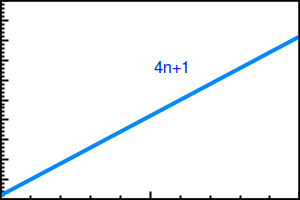In all curve graphs that will be represented in this book, the x axis represents Input Size (n) and the y axis represents Execution Time.

As we can see in the preceding graph, the curve is linear. However, since the time complexity also depends on the other parameters, such as hardware specification, we may have another complexity for the preceding `Looping()` function if we run the function on faster hardware. Let's say the time complexity becomes 2n + 0.5, so that the curve will be as follow: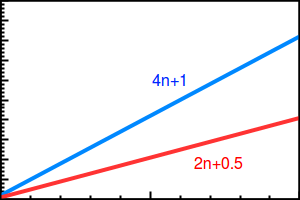As we can see, the curve is still linear for the two complexities. For this reason, we can omit a constant and the least significant parts in asymptotic analysis, so we can say that the preceding complexity is `n`, as found in the following notation:

`Time Complexity(n) = n`

Let's move on to another function. If we have the nested `while` loop, we will have another complexity, as we can see in the following code:

```void Pairing(int n)
{
int i = 0;

while(i < n)
{
int j = 0;

while(j < n)
{
cout << i << ", " << j << endl;
j = j + 1;
}
i = i + 1;
}
}```

Based on the preceding `Looping()` function, we can say that the complexity of the inner `while` loop of the preceding `Pairing()` function is `4n + 1`. We then calculate the outer `while` loop so it becomes `1 + (n * (1 + (4n + 1) + 2)`, which equals `1 + 3n + 4n2`. Therefore, the complexity of the preceding code is:

`Time Complexity(n) = 4n2 + 3n + 1`

The curve for the preceding complexity will be as follows: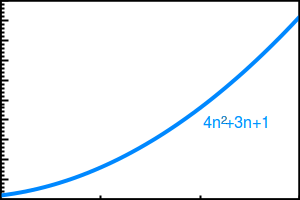And if we run the preceding code in the slower hardware, the complexity might become twice as slow. The notation should be as follows:

`Time Complexity(n) = 8n2 + 6n + 2`

And the curve of the preceding notation should be as follows:As shown previously, since the asymptotic analysis omits the constants and the least significant parts, the complexity notation will be as follows:

`Time Complexity(n) = n2`

### Worst, average, and best cases

In the previous section, we were able to define time complexity for our code using an asymptotic algorithm. In this section, we are going to determine a case of the implementation of an algorithm. There are three cases when implementing time complexity in an algorithm; they are worst, average, and best cases. Before we go through them, let's look at the following `Search()` function implementation:

```int Search(int arr[], int arrSize, int x)
{
// Iterate through arr elements
for (int i = 0; i < arrSize; ++i)
{
// If x is found
// returns index of x
if (arr[i] == x)
return i;
}

// If x is not found
// returns -1
return -1;
}```

As we can see in the preceding `Search()` function, it will find an index of target element (`x`) from an `arr` array containing `arrSize` elements. Suppose we have the array `{42, 55, 39, 71, 20, 18, 6, 84}`. Here are the cases we will find:

• Worst case analysis is a calculation of the upper bound on the running time of the algorithm. In the `Search()` function, the upper bound can be an element that does not appear in the `arr`, for instance, `60`, so it has to iterate through all elements of `arr` and still cannot find the element.
• Average case analysis is a calculation of all possible inputs on the running time of algorithm, including an element that is not present in the `arr` array.
• Best case analysis is a calculation of the lower bound on the running time of the algorithm. In the `Search()` function, the lower bound is the first element of the `arr` array, which is `42`. When we search for element `42`, the function will only iterate through the `arr` array once, so the `arrSize` doesn't matter.

### Big Theta, Big-O, and Big Omega

After discussing asymptotic analysis and the three cases in algorithms, let's discuss asymptotic notation to represent the time complexity of an algorithm. There are three asymptotic notations that are mostly used in an algorithm; they are Big Theta, Big-O, and Big Omega.

The Big Theta notation (θ) is a notation that bounds a function from above and below, like we saw previously in asymptotic analysis, which also omits a constant from a notation.

Suppose we have a function with time complexity `4n + 1`. Since it's a linear function, we can notate it like in the following code:

`f(n) = 4n + 1`

Now, suppose we have a function, `g(n)`, where `f(n)` is the Big-Theta of `g(n)` if the value, `f(n)`, is always between `c1*g(n) (lower bound)` and `c2*g(n) (upper bound)`. Since `f(n)` has a constant of `4` in the n variable, we will take a random lower bound which is lower than `4`, that is `3`, and an upper bound which is greater than `4`, that is `5`. Please see the following curve for reference: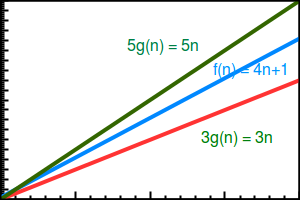From the `f(n)` time complexity, we can get the asymptotic complexity `n`, so then we have `g(n) = n`, which is based on the asymptotic complexity of `4n + 1`. Now, we can decide the upper bound and lower bound for `g(n) = n`. Let's pick `3` for the lower bound and `5` for the upper bound. Now, we can manipulate the `g(n) = n` function as follows:

```3.g(n) = 3.n
5.g(n) = 5.n```

Big-O notation (O) is a notation that bounds a function from above only using the upper bound of an algorithm. From the previous notation, `f(n) = 4n + 1`, we can say that the time complexity of the `f(n)` is `O(n)`. If we are going to use Big Theta notation, we can say that the worst case time complexity of `f(n)` is `θ(n)` and the best case time complexity of `f(n)` is `θ(1)`.

Big Omega notation is contrary to Big-O notation. It uses the lower bound to analyze time complexity. In other words, it uses the best case in Big Theta notation. For the `f(n)` notation, we can say that the time complexity is `Ω(1)`

### Recursive method

In the previous code sample, we calculated the complexity of the iterative method. Now, let's do this with the recursive method. Suppose we need to calculate the factorial of a certain number, for instance `6`, which will produce `6 * 5 * 4 * 3 * 2 * 1 = 720`. For this purpose, we can use the recursive method, which is shown in the following code snippet:

```int Factorial(int n)
{
if(n == 1)
return 1;

return n * Factorial(n - 1);
}```

For the preceding function, we can calculate the complexity similarly to how we did for the iterative method, which is `f(n) = n` since it depends on how much data is being processed (which is `n`). We can use a constant, for instance `c`, to calculate the lower bound and the upper bound.

### Amortized analysis

In the previous section, we just discussed the single input, `n`, for calculating the complexity. However, sometimes we will deal with more than just one input. Please look at the following `SumOfDivision()` function implementation:

```int SumOfDivision(
int nArr[], int n, int mArr[], int m)
{
int total = 0;

for(int i = 0; i < n; ++i)
{
for(int j = 0; j < m; ++j)
{
total += (nArr[i] * mArr[j]);
}
}

return total;
}```

And that's where amortized analysis comes in. Amortized analysis calculates the complexity of performing the operation for varying inputs, for instance, when we insert some elements into several arrays. Now, the complexity doesn't only depend on the `n` input only, but also the `m` input. The complexity can be as follows:

`Time Complexity(n, m) = n · m`

We are going to discuss these analysis methods in more detail in the following chapters.

## Summary

This chapter provided us with an introduction to basic C++ (simple program, IDE, flow control) and all data types (fundamental and advanced data types, including template and STL) that we will use to construct the data structures in the following chapters. We also discussed a basic complexity analysis, and we will dig into this deeper in the upcoming chapters.

Next, we are going to create our first data structures, that is, linked list, and we are going to perform some operations on the data structure.

## QA section

• What is the first function in C++ which is executed for the first time?
• Please list the fundamental data types in C++!
• What can we use to control the flow of code?
• What is the difference between enums and structs?
• What are the abstraction, information hiding, and encapsulation techniques? Please explain!
• What is the keyword to create a template in C++?
• What is the difference between the function template and the class template?
• What is the difference between Big Theta, Big-O, and Big Omega?

## Further reading

You can also refer to the following links:

### About the Author

• ##### Wisnu Anggoro

Wisnu Anggoro is a Microsoft Certified Professional in C# programming and an experienced C/C++ developer. He has also authored the books Boost.Asio C++ Network Programming - Second Edition and Functional C# by Packt. He has been programming since he was in junior high school, which was about 20 years ago, and started developing computer applications using the BASIC programming language in the MS-DOS environment. He has solid experience in smart card programming, as well as desktop and web application programming, including designing, developing, and supporting the use of applications for SIM Card Operating System Porting, personalization, PC/SC communication, and other smart card applications that require the use of C# and C/C++.

He is currently a senior smart card software engineer at CIPTA, an Indonesian company that specializes in innovation and technology for smart cards. He can be reached through his email at [email protected]

Browse publications by this author

### Latest Reviews

(7 reviews total)